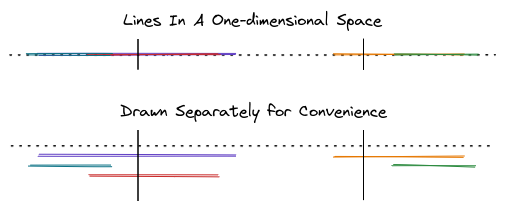# One-dimensionalTough Dash, June 2023
Limits 1s, 512 MB

Imagine there are several lines in an one dimensional space. You are given the starting and ending points of all of these lines.Given another pair of points, you have to determine the number of lines that goes through that region.

In the diagram you can see that the purple, red and orange lines are visible between the two vertical black lines (boundary). In this scenario the answer would be 3.

A line falls within the boundary of the two vertical lines even if it touches the point. For example, if the ending point of a line touches the starting boundary the line is considered to have fallen within the boundary.

## Input

The input will start with two integers $N$ ($1 \le N \le 1000$) and $M$ ($1 \le M \le 1000$). $N$ is the number of lines and $M$ is the number of queries.

Next $N$ lines will follow. Each line will have a pair of 32-bit signed integers. These pair of integers represent the starting point and ending point of a line.

Next $M$ lines will follow. Each line will have a pair of 32-bit signed integers. These pair of integers represent the starting point and ending point of the boundary. You will have to determine how many lines fall within this boundary as described in the problem statement.

## Output

For each of the $M$ queries you have to print the number of lines that has fallen within the given boundary.

## Sample

InputOutput
5 1
-3 3
-4 -2
-2 2
8 12
12 14
0 10

3


This sample test case has been visualized roughly in the problem statement.

### Submit